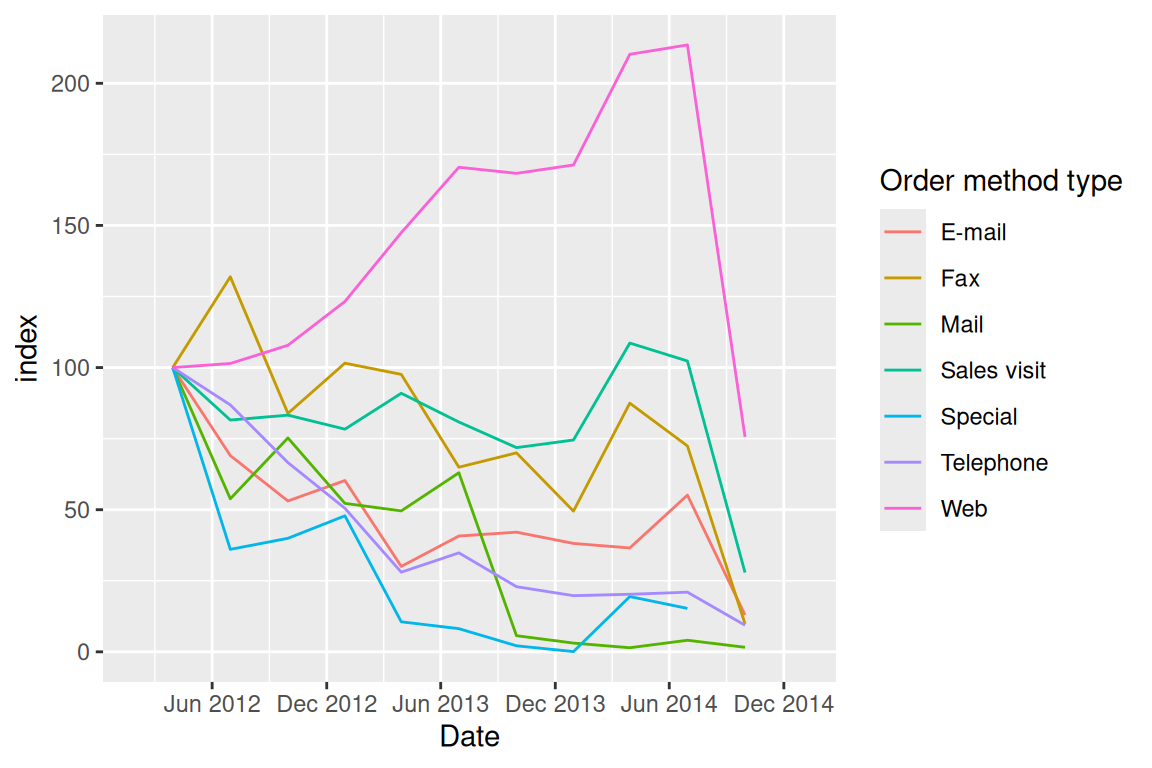# Chapter 16 Time series

Time series, by definition, is a sequence of data point collected over a certain period of time. In this chapter, we will demonstrate several useful ways of plotting time-series data and how to processing `date` data type in R.

## 16.1 Dates

Since time series analysis looks into how data is changing over time, the very first step is to transform the data into correct format.

### 16.1.1 Basic R functions

You can convert character data to `Date` class with `as.Date()`:

``````dchar <- "2018-10-12"
ddate <- as.Date(dchar)
class(dchar)``````
``##  "character"``
``class(ddate)``
``##  "Date"``

You can also specifying the format by:

``as.Date("Thursday, January 6, 2005", format = "%A, %B %d, %Y")``
``##  "2005-01-06"``

For a list of the conversion specifications available in R, see `?strptime`.

Here is a list of the conversion specifications for date format from this postAlso, `Date` class supports calculation between dates:

``as.Date("2017-11-02") - as.Date("2017-01-01")``
``## Time difference of 305 days``
``as.Date("2017-11-12") > as.Date("2017-3-3")``
``##  TRUE``

### 16.1.2 Lubridate

The tidyverse `lubridate` makes it easy to convert dates that are not in standard format with `ymd()`, `ydm()`, `mdy()`, `myd()`, `dmy()`, and `dym()` (among many other useful date-time functions):

``lubridate::mdy("April 13, 1907")``
``##  "1907-04-13"``

The `lubridate` package also provides additional functions to extract information from a date:

``````today <- Sys.Date()
lubridate::year(today)``````
``##  2022``
``lubridate::yday(today)``
``##  95``
``lubridate::month(today, label = TRUE)``
``````##  Apr
## 12 Levels: Jan < Feb < Mar < Apr < May < Jun < Jul < Aug < Sep < ... < Dec``````
``lubridate::week(today)``
``##  14``

## 16.2 Time series

For time-series data-sets, line plots are mostly used with time on the x-axis. Both base R graphics and `ggplot2` “know” how to work with a Date class variable, and label the axes properly:

The data comes from the official website.

``````library(dplyr)
library(tidyr)
library(ggplot2)
col_types = c("date", "numeric", "numeric",
"numeric"))

plot(df\$Week, df\$`30 yr FRM`, type = "l") # on the order of years``````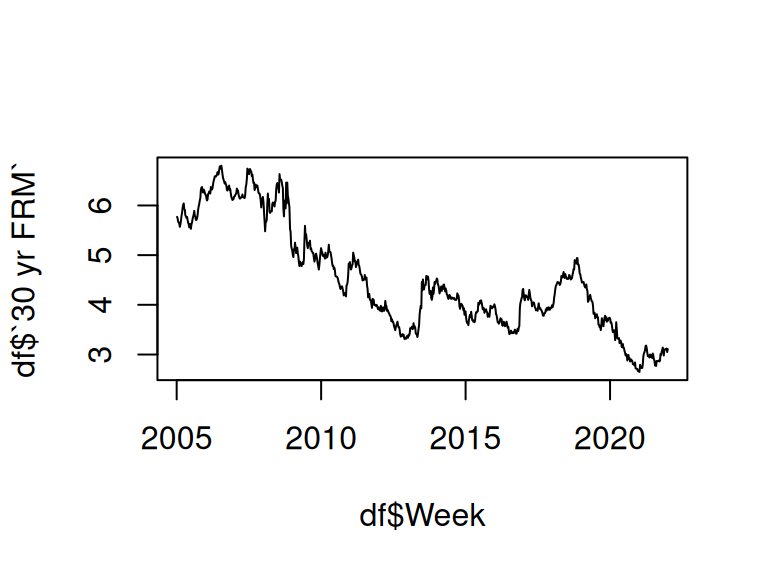``````g<-ggplot(df %>% filter(Week < as.Date("2006-01-01")),
aes(Week, `30 yr FRM`)) +
geom_line() +
theme_grey(14)
g``````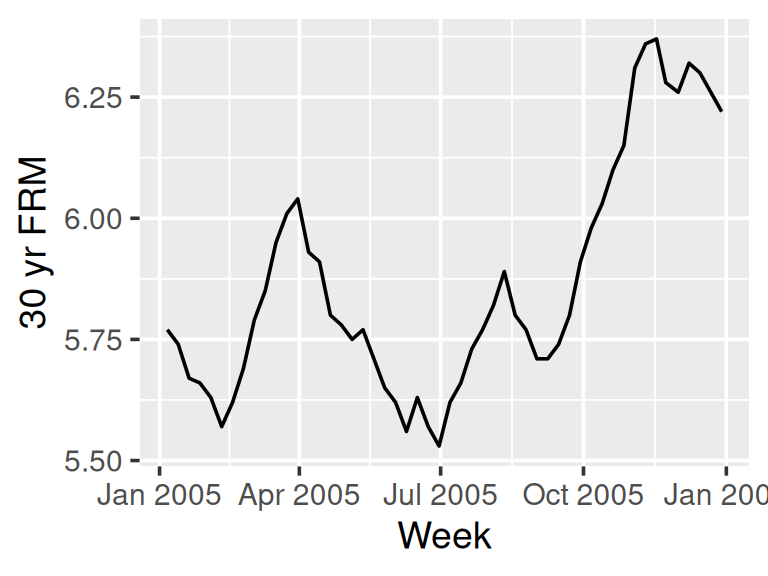We can control the x-axis breaks, limits, and labels with `scale_x_date()`, and use `geom_vline()` with `annotate()` to mark specific events in a time series.

## 16.3 Multiple time series

The following plot shows a multiple time series of U.S. Mortgage rates.

``````df2 <- df %>% pivot_longer(cols = -c("Week"), names_to = "TYPE") %>%
mutate(TYPE = forcats::fct_reorder2(TYPE, Week, value))# puts legend in correct order

ggplot(df2, aes(Week, value, color = TYPE)) +
geom_line() +
ggtitle("U.S. Mortgage Rates") +  labs (x = "", y = "percent") +
theme_grey(16) +
theme(legend.title = element_blank())``````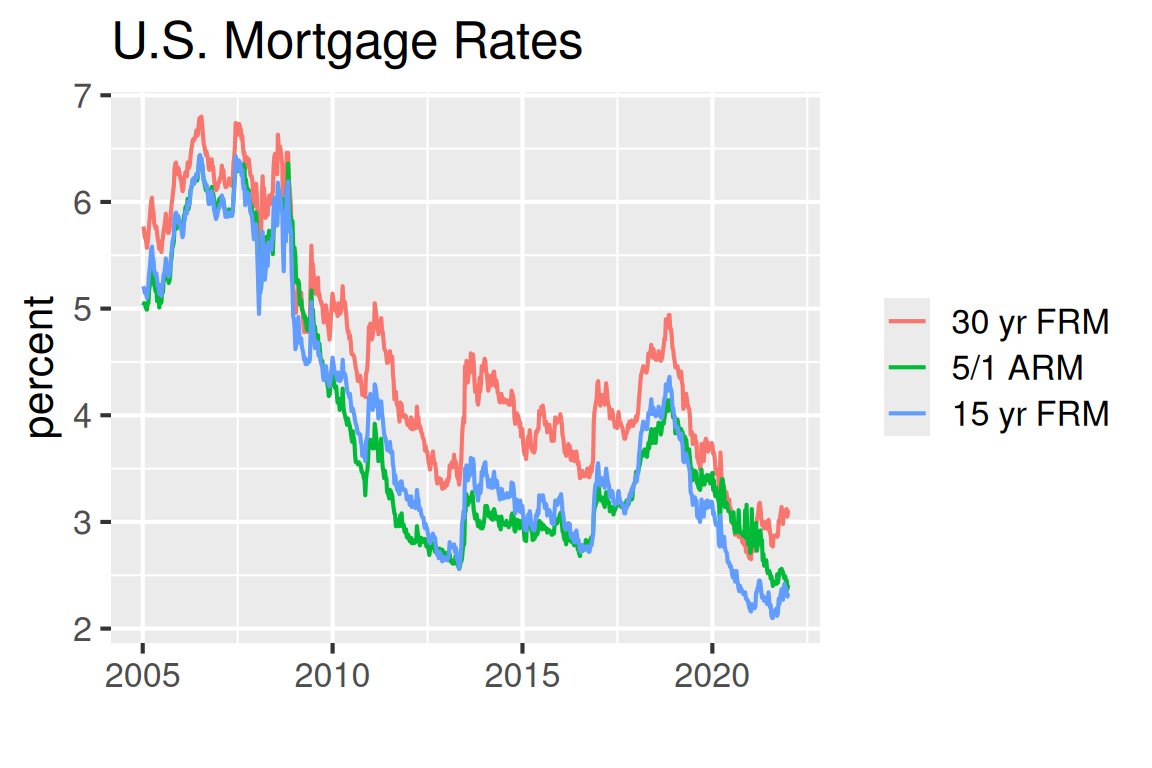To plot the time series in a specific period of time, use `filter()` before `ggplot`:

``````library(lubridate)
df2010 <- df2 %>% filter(year(Week) == 2010)
ggplot(df2010, aes(Week, value, color = TYPE)) +
geom_line() +
ggtitle("U.S. Mortgage Rates")``````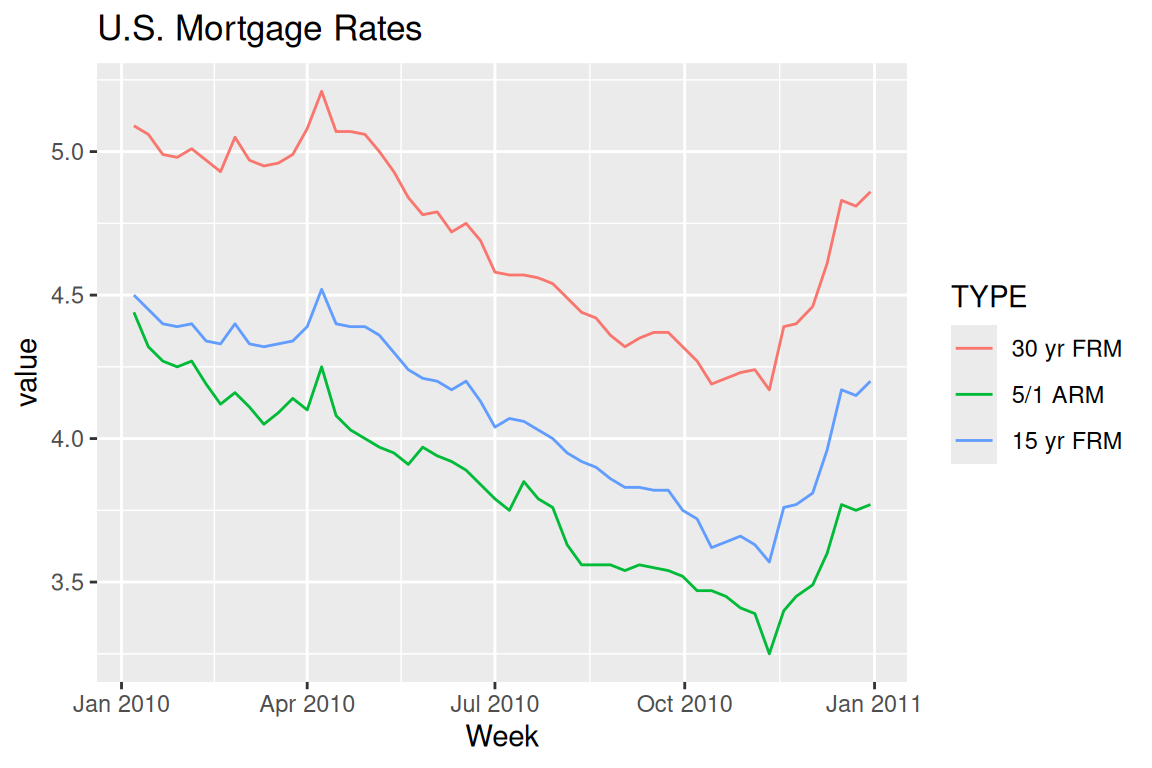## 16.4 Time series patterns

Next, as an important part of time series analysis, we want to find the existing patterns of the data. We first starting from plotting the overall long-term trend:

``````library (readr)
urlfile="https://raw.githubusercontent.com/jtr13/data/master/ManchesterByTheSea.csv"

g <- ggplot(data, aes(Date, Gross)) +
geom_line() +
ggtitle("Manchester by the Sea", "Daily Gross (US\$), United States") +
xlab("2016-2017")
g``````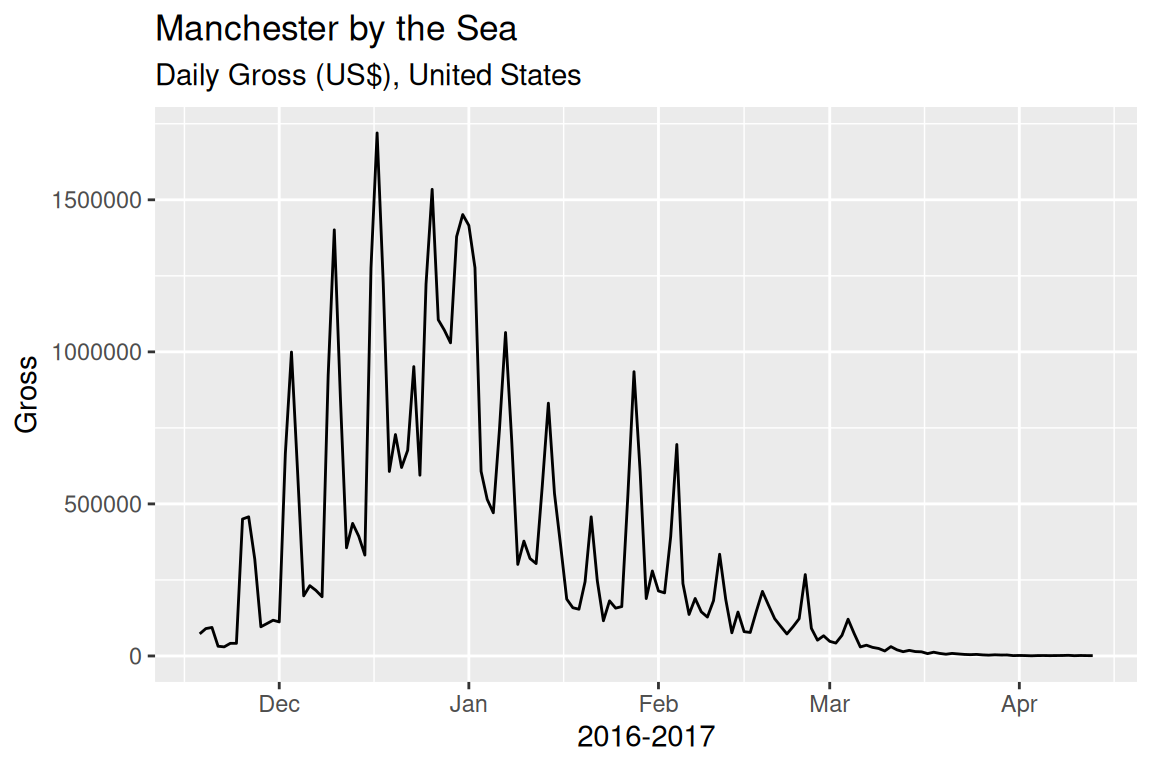Adding a smoother to the data, adjusting the smoothing parameter `span =` to find a proper smoother which is not overfitting/underfitting:

``````g <- ggplot(data, aes(Date, Gross)) + geom_point()
g + geom_smooth(method = "loess", span = .5, se = FALSE)``````Mark the pattern by high-lighting the data on very Saturday:

``````g <- ggplot(data, aes(Date, Gross)) +
geom_line() +
ggtitle("Manchester by the Sea", "Daily Gross, United States")
saturday <- data %>% filter(wday(Date) == 7)
g +
geom_point(data = saturday, aes(Date, Gross), color = "deeppink")``````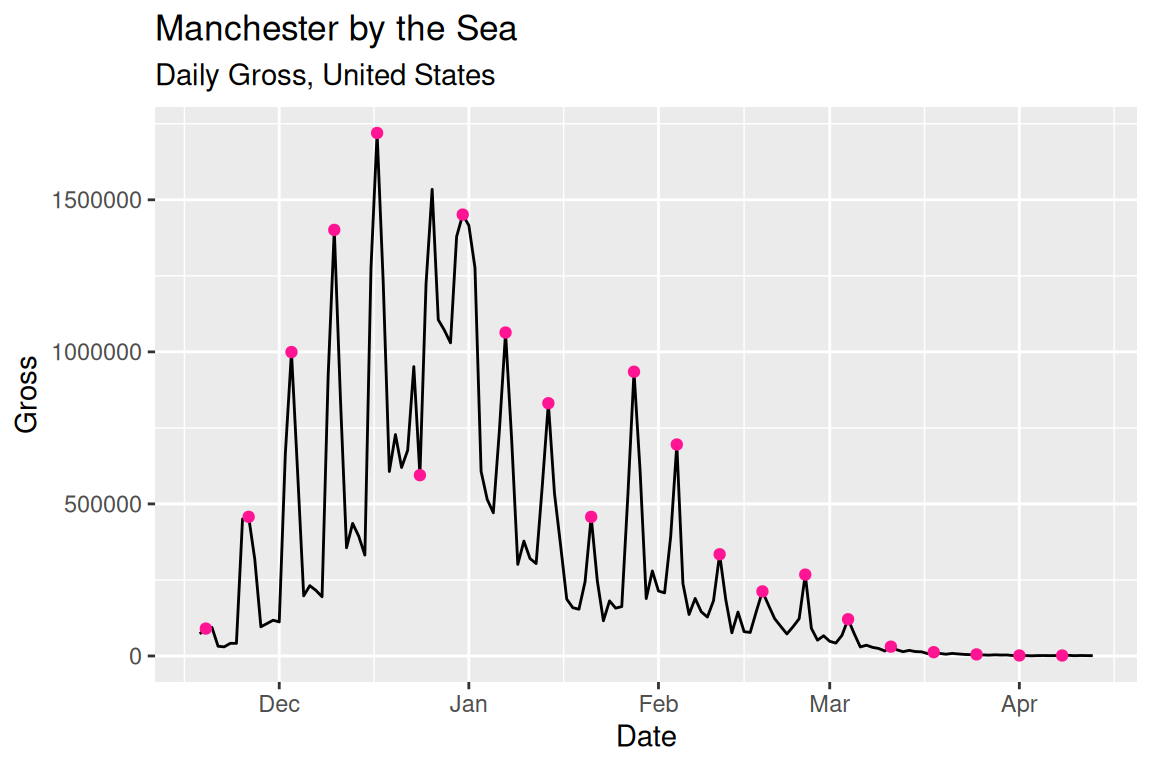Another way is to use facet to show the cyclical pattern:

``````ggplot(data, aes(Date, Gross)) +
geom_line(color = "grey30") + geom_point(size = 1) +
facet_grid(.~wday(Date, label = TRUE)) +
geom_smooth(se = FALSE)``````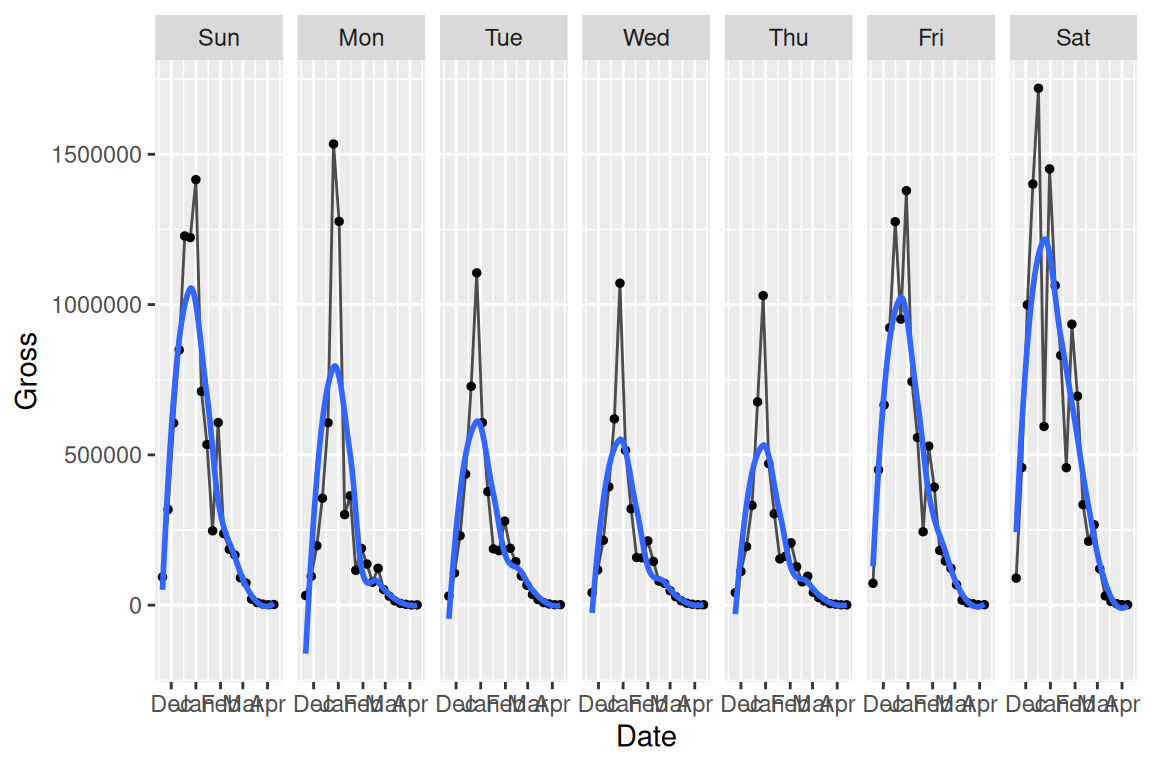Also, basic R can plot the decomposed time series automatically. This method is used to study the trend, seasonal effect on data with at least 2 periods. For additive components, use `type = "additive"`.

``````tsData <- EuStockMarkets[, 2]
decomposedRes <- decompose(tsData, type="mul")
plot (decomposedRes)``````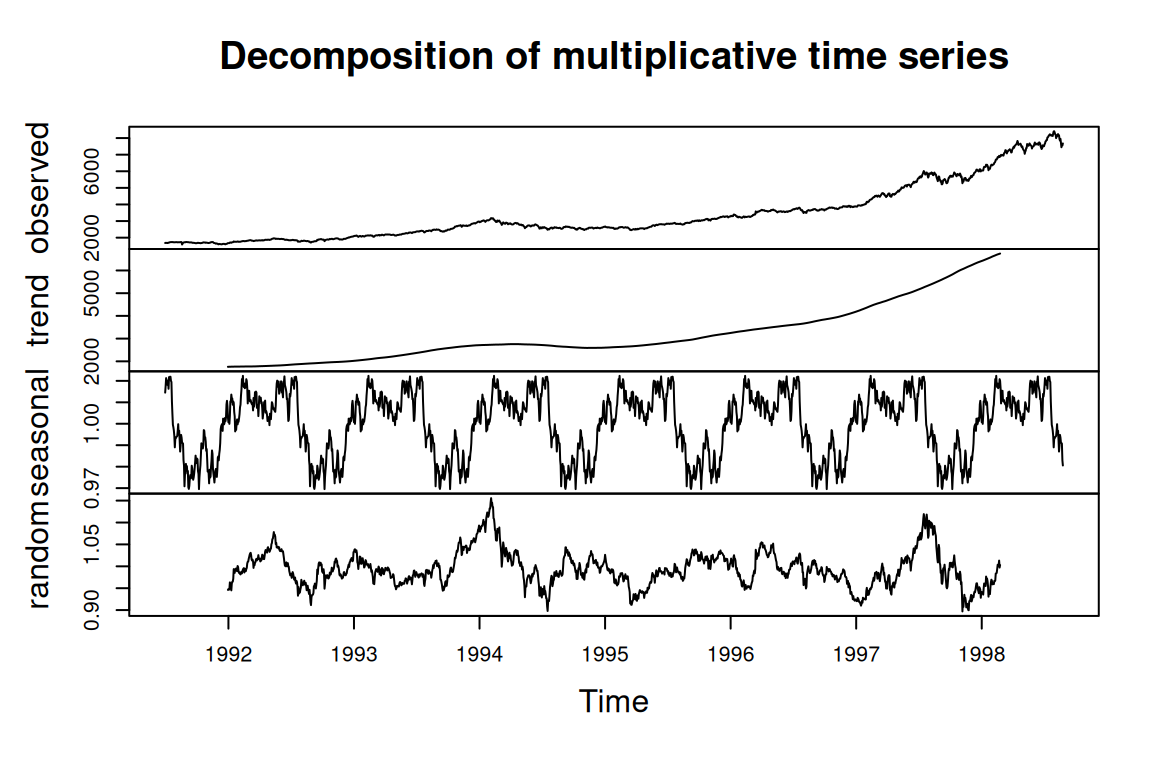## 16.5 Index

When making comparisons on multi-line plots, index is a way of scaling the data: Each value is divided by the first value for that group and multiplied by 100.

``````urlfile="https://raw.githubusercontent.com/jtr13/data/master/WA_Sales_Products_2012-14.csv"

sale\$Q <- as.numeric(substr(sale\$Quarter, 2, 2))
# convert Q to end-of-quarter date
sale\$Date <- as.Date(paste0(sale\$Year, "-",as.character(sale\$Q*3),"-30"))

Methoddata <- sale %>%
mutate(Revenue = Revenue/1000000) %>%
group_by(Date,`Order method type`) %>%
summarize(Revenue = sum(Revenue))  %>%
ungroup() %>%
group_by(`Order method type`) %>%
mutate(index = round(100*Revenue/Revenue, 2)) %>%
ungroup()

g <- ggplot(Methoddata, aes(Date, index,color = `Order method type`)) +
geom_line(aes(group = `Order method type`)) +
scale_x_date(limits = c(as.Date("2012-02-01"), as.Date("2014-12-31")), date_breaks = "6 months", date_labels = "%b %Y")
g``````